The EllipseCustom SearchTHE ELLIPSE An ellipse is a conic section with an eccentricity greater than 0 and less than 1. Referring to figure 2-11, let PO =a FO =c OM = d where F is the focus, O is the center, and P and P' are points on the ellipse. Then from the definition of eccentricity,Figure 2-11.-Development of focus and directrix.and,Subtraction and addition of the two equations givePlace the center of the ellipse at the origin so that one focus lies at ( - ae,0) and one directrix is the line x = - ale. Figure 2-12 shows a point on the Y axis that satisfies the conditions for an ellipse. If P"O=b and FO =c then By definition, e is the ratio of the distance of P" from the focus and the directrix, so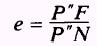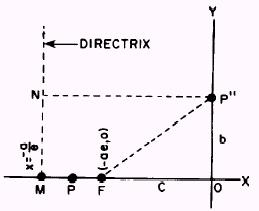Figure 2-12.-Focus, directrix, and point P".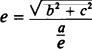or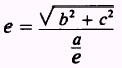Multiplying both sides by ale gives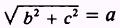orso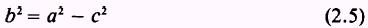Now combining equations (2.4) and (2.5) givesor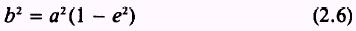Refer to figure 2-13. If the point (x,y) is on the ellipse, then the ratio of its distance from F to its distance from the directrix is e. The distance from (x,y) to the focus ( - ae,0) isand the distance from (x,y) to the directrix x =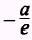isThe ratio of these two distances is equal to e, so (x + ae)2 + y2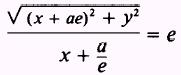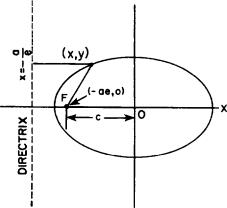Figure 2-13.-The ellipse.   or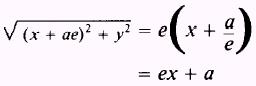Squaring and expanding both sides gives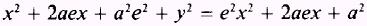Canceling like terms and transposing terms in x to the left-hand side of the equation givesRemoving a common factor givesDividing both sides of equation (2.7) by the right-hand member givesFrom equation (2.6) we obtain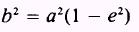so that the equation becomesThis is the equation of an ellipse in standard form. In figure 2-14, views A and B, a is the length of the semimajor axis and b is the length of the semimanor axis. The curve is symmetrical with respect to the X and Y axes, so you can easily see that figure 2-14, view A, has another focus at (ae,0) and a corresponding directrix, x = a/e. The curve also has vertices at ( � a,0). The distance from the center through the focus to the curve is always designated a and is called the semimajor axis. This axis may be in either the x or y direction. When it is in the y direction, the directrix is a line denoted by the equation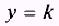Figure 2-14.-Ellipse showing axes. In the case we have studied, the directrix was denoted by the formulawhere k is a constant equal to � a/e. The perpendicular distance from the midpoint of the major axis to the curve is called the semiminor axis and is always signified by b. The distance from the center of the ellipse to the focus is called c. In any ellipse the following relations are true for a, b, and c: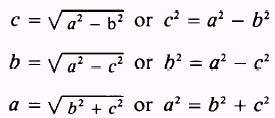Whenever the directrix is a line denoted by the equation y = k, the major axis is in the y direction and the equation of the ellipse is as follows: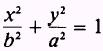Refer to figure 2-14, view B. This curve has foci at (0, � c) and vertices at (0, � a). In an ellipse the position of the a2 and b2 terms indicates the orientation of the ellipse axis. As shown in figure 2-14, views A and B, value a is the semimajor or longer axis. In the previous paragraphs formulas were given showing the relationship between a, b, and c. In the first portion of this discus�sion, a formula showing the relationship between a, c, and the eccentricity was given. These relationships are used to find the equation of an ellipse in the following example: EXAMPLE: Find the equation of the ellipse with its center at the origin and having foci atand an eccentricity equal to, SOLUTION: With the focal points on the X axis, the ellipse is oriented as in figure 2-14, view A, and the standard form of the equation is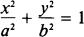With the center at the origin, the numerators of the fractions on the left are x2 and y2. The problem is to find the values of a and b. The distance from the center to either of the foci is equal to c (fig. 2-14, view A), so in this problem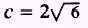from the given coordinates of the foci. The values of a, c, and e (eccentricity) are related by c=ae or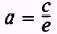From the known information, substitute the values of c and e,andThen, using the formulaorand substituting for a2 and c2,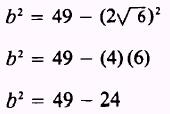gives the final required value of b2 = 25 Then the equation of the ellipse is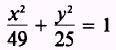Integrated Publishing, Inc. - A (SDVOSB) Service Disabled Veteran Owned Small Business